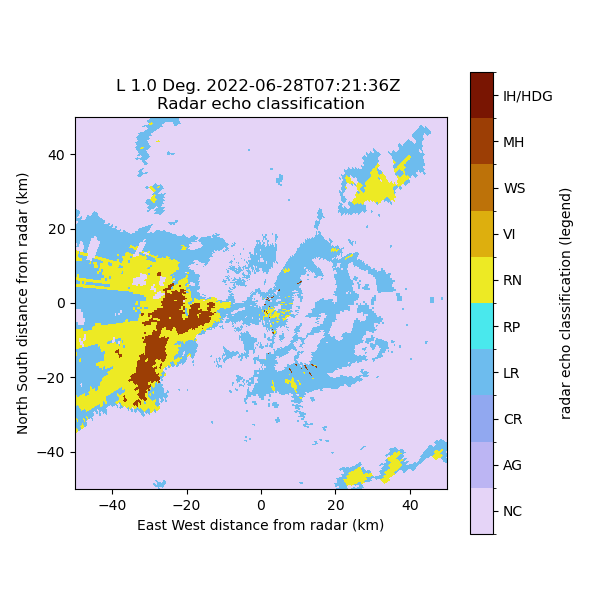# Calculate and Plot hydrometeor classification#

Calculates a hydrometeor classification and displays the results```# Author: Daniel Wolfensberger (daniel.wolfensberger@meteoswiss.ch)

import matplotlib as mpl
import matplotlib.pyplot as plt
import numpy as np

import pyart

# Read in a sample file
filename = DATASETS.fetch("MLL2217907250U.003.nc")

# Read temperature preinterpolated from NWP model
filename = DATASETS.fetch("20220628072500_savevol_COSMO_LOOKUP_TEMP.nc")

# Compute attenuation
out = pyart.correct.calculate_attenuation_zphi(
phidp_field="uncorrected_differential_phase",
temp_field="temperature",
temp_ref="temperature",
)
spec_at, pia, cor_z, spec_diff_at, pida, cor_zdr = out

# Compute KDP
kdp, _, _ = pyart.retrieve.kdp_maesaka(
)

# Compute hydrometeor classification
hydro = pyart.retrieve.hydroclass_semisupervised(
refl_field="corrected_reflectivity",
zdr_field="corrected_differential_reflectivity",
kdp_field="specific_differential_phase",
rhv_field="uncorrected_cross_correlation_ratio",
temp_field="temperature",
)

# Display hydrometeor classification with categorical colormap
fig, ax = plt.subplots(1, 1, figsize=(6, 6))

labels = ["NC", "AG", "CR", "LR", "RP", "RN", "VI", "WS", "MH", "IH/HDG"]
ticks = np.arange(len(labels))
boundaries = np.arange(-0.5, len(labels))
norm = mpl.colors.BoundaryNorm(boundaries, 256)

cax = display.plot_ppi(
"radar_echo_classification", 0, ax=ax, norm=norm, ticks=ticks, ticklabs=labels
)

ax.set_xlim([-50, 50])
ax.set_ylim([-50, 50])
ax.set_aspect("equal", "box")

# For info
# NC = not classified
# AG = aggregates
# CR = ice crystals
# LR = light rain
# RP = rimed particles
# RN = rain
# VI = vertically oriented ice
# WS = wet snow
# MH = melting hail
# IH/HDG = dry hail / high density graupel
```

Total running time of the script: ( 0 minutes 3.969 seconds)

Gallery generated by Sphinx-Gallery